Motion

If you go at speed 3.7 km/h, you come to the station 42 minutes after leaving train. If you go by bike to the station at speed 27 km/h, you come to the station 56 minutes before its departure.
How far is the train station?

Result

s =  7.003 km

Solution:Leave us a comment of this math problem and its solution (i.e. if it is still somewhat unclear...):Be the first to comment!To solve this verbal math problem are needed these knowledge from mathematics:

Do you have a linear equation or system of equations and looking for its solution? Or do you have quadratic equation? Do you want to convert length units?

Next similar examples:

1. Mail trainThe speed of mail train is 1370 meter per minute. Express it in miles per hour correct to three significant figure . Given that 1 meter =39.37 inches.
2. Two trainsThere were 159 freight wagons on the railway station creating 2 trains. One had 15 more wagons than the other. How many wagons did each train have?
3. After 16 years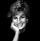After 16 years will Dana be five times older as she was four years ago. After how many years will Dana celebrate her 16th birthday?
4. SuzanSusan's age will be after 12 years four times as much as twelve years ago. How old is Susan now?
5. Book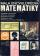To number pages of thick book was used 4201 digits. How many pages has this book?
6. School trip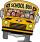School trip cost 247.2 Eur for one class (24 students). How much would cost a trip for two classes? (both classes together have 53 students)
7. Log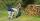Worker cut his thick log to 6 pieces for 30 min. How long he cut log to 12 pieces?
8. CakesGrandmother baked cakes. Half of its was poppy, quarter with plum jam and 16 cheesecakes. How many cakes she baked in total?
9. Number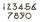What number I think? The third is 6 and half is 2 more than the its quarter.
10. The classroom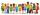In the seventh class the number of boys and girls are at a ratio of 4:5. Boys are 16. How many total students are in the classroom?
11. Unknown numberIdentify unknown number which 1/5 is 40 greater than one tenth of that number.
12. Tippler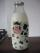Bottle with cork cost 8.8 Eur. The bottle is 0.8 euros more expensive than cork. How much is a bottle and the cork?
13. Father and sonWhen I was 11, my father was 35 years old. Today, my father has three times more years than me. How old is she?
14. 1.5 divided1.5 divided by 1 = w divided by 4
15. Linear systemSolve this linear system (two linear equations with two unknowns): x+y =36 19x+22y=720
16. Negative in equation2x + 3 + 7x = – 24, what is the value of x?
17. Bed timeTiffany was 5 years old; her week night bedtime grew by ¼ hour each year. If, at age 18, her curfew time is 11pm, what was her bed time when she was 5 years old?